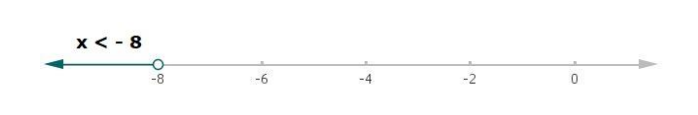# Solve each of the following in equations and represent the solution set onQuestion:

Solve each of the following in equations and represent the solution set on the number line.

$\frac{5 x}{4}-\frac{4 x-1}{3}>1$, where $x \in \mathbf{R}$

Solution:

Given:

$\frac{5 x}{4}-\frac{4 x-1}{3}>1$, where $x \in R$

$\frac{3(5 x)-4(4 x-1)}{12}>1$

$\frac{15 x-16 x+4}{12}>1$

$\frac{-x+4}{12}>1$

Now, multiplying by 12 on both the sides in the above equation,

$\left(\frac{-x+4}{12}\right) \cdot(12)>1$. (12)

$-x+4>12$

Now, subtracting 4 from both the sides in above equation

$-x+4-4>12-4$

$-x>8$

Multiplying by -1 on both the sides of the above equation

$x<-8$